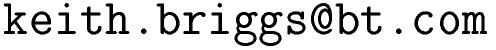Keith Briggshome · papers · thesis · talks · meetings · records · maths notes « · software · languages · music · travel · cv · students · maps · place-names · people · photos · links · ex libris · site map### Independence and correlation

by Seb Wills
```Definitions:

y_1 and y_2 are uncorrelated means E{y_1 y_2} - E{y_1}E{y_2} = 0

y_1 and y_2 are independent means p(y_1,y_2) = p_1(y_1) p_2(y_2), i.e.
the joint pdf is factorisable. (Intuitively, this means that learning the
value of one of the variables doesn't tell you anything about the value of
the other).

Fact:
Independence implies uncorrelatedness, but the reverse is not true.

Proof that two random variables can be uncorrelated but not independent:
(based on Aapo Hyvarinen's paper "Independent Component Analysis: A
Tutorial", at
http://www.cis.hut.fi/aapo/papers/IJCNN99_tutorialweb/node7.html)

It is easy to show that for any independent variables y_1 and y_2,

E{h_1(y_1) h_2(y_2)} = E{h_1(y_1)} E{h_2(y_2)} for any functions h_1 and h_2

(property M)

where E{} denotes expectation.

Consider (y_1,y_2) are discrete and have a joint distribution such that
the following (y_1,y_2) pairs are equally likely:
(0,1),(0,-1),(1,0),(-1,0). It is easy to verify that they are
uncorrelated. But if we choose h_1 and h_2 to be the squared function,
then the above property, M, is violated. Since independence implies M
holds for all functions, and we have found a function for which it does
not hold, y_1 and y_2 cannot be independent.

Since the logic can be confusing, here it is in detail:

Independent => M for all h_1, h_2
therefore
not(M for all h_1,h_2) => not(Independent)
therefore
there exists an h_1, h_2 for which M not true => not(Independent)

It is also easy to see intuitively that the above counter-example works:
the marginalised pdf of y_1 is that it takes the values {-1,0,1} with
probabilities {1/4, 1/2, 1/4}. But if we know that y_2 is -1, then that
constrains y_1 to be zero. So knowing y_2 has changed our knowledge of
y_1.

Another counterexample:

Let s and a be discrete random variables which take values -1 and 1
with 50% probability each.

Let x take the value of a if s=1, otherwise zero. Now x and s are clearly
uncorrelated, but are not independent since knowing s determines the pdf
of x.
```
This website uses no cookies. This page was last modified 2013-05-12 10:17 by.